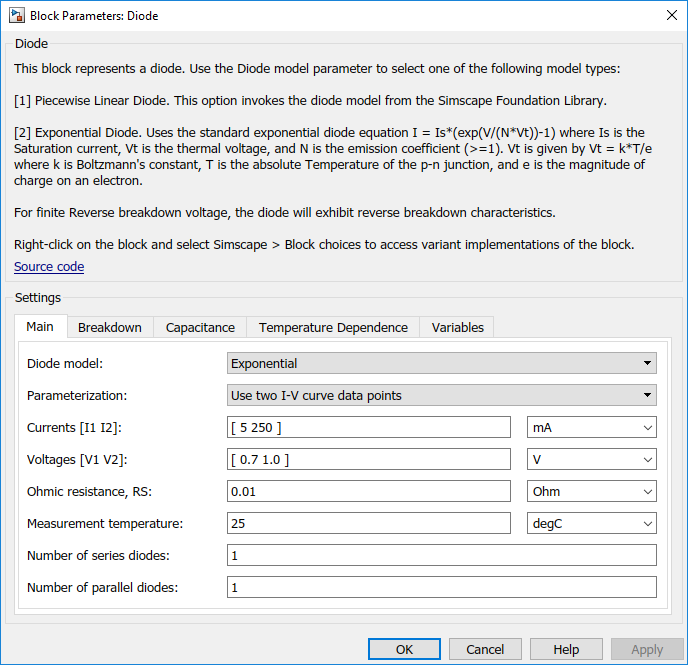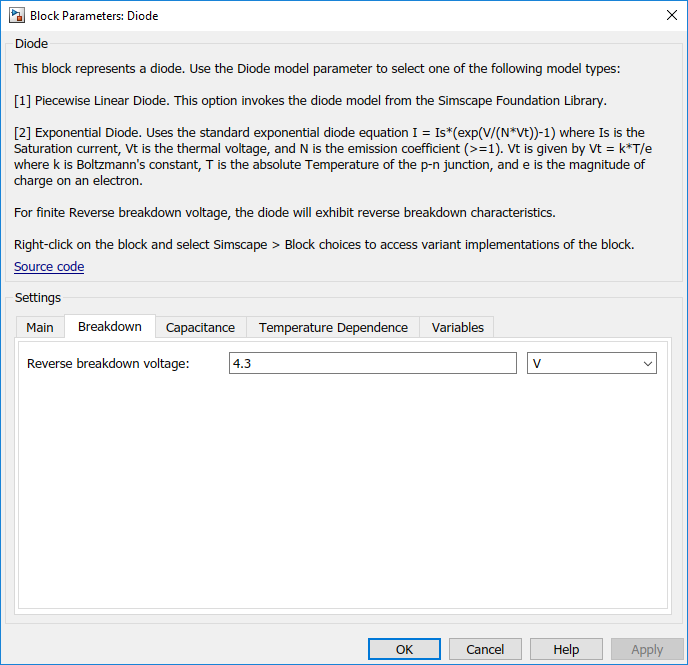## Parameterize an Exponential Diode from a Datasheet

Example 1 uses a piecewise linear approximation to the diode’s exponential current-voltage relationship. This results in more efficient simulation, but requires some thought to go into the setting of block parameter values. An alternative is to use a more complex model that is valid for a wider range of voltage and current values. This example uses the `Exponential` parameterization option of the Diode block.

This model either requires two data points from the diode current-voltage relationship, or values for the underlying equation coefficients, namely the saturation current IS and the emission coefficient N. The BZX384-B4V3 datasheet only provides values for the former case. Some datasheets do not give the necessary data for either case, and you must follow the processes in Example 1 or Example 3 instead.

The two data points in the table below are from the BZX384-B4V3 datasheet current-voltage curve:

 Diode forward voltage, VF 0.7V 1V Diode forward current, IF 5mA 250mA

Set the exponential diode block parameters as follows:

• Currents [I1 I2]. Set to [5 250] mA.

• Voltages [V1 V2]. Set to [0.7 1.0] V.

• Reverse breakdown voltage. Set to the datasheet working voltage value, 4.3V.

• Ohmic resistance, RS. Set to 0.01 Ω. This is an example of a parameter that cannot be determined from the datasheet. However, setting its value to zero is not necessarily a good idea, because a small value can help simulation convergence for some circuit topologies. Physically, this term will not be zero because of the connection resistances.

• Junction capacitance. Set to the datasheet diode capacitance value, 450 pF.

A more complex capacitance model is also available for the Diode component with the exponential equation option. However, the datasheet does not provide the necessary data. Moreover, the operation of this circuit is not sufficiently sensitive to voltage-dependent capacitance effects to warrant the extra detail.